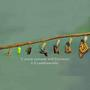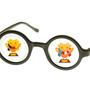1. Forum
2. >
3. Topic: German
4. >
5. "Zwei plus zwei gleich vier."

# "Zweipluszweigleichvier."

## Translation:Two plus two equals four.

October 13, 2015•6

2 + 2 = 4 will not be accepted?•567

German --> English

NOT

German --> German

Numbers are also German•2350

"2 + 2 = 4" is now accepted as correct, however, I agree with the others who have indicated that Duo users would likely learn more if it were not accepted.So you'll actually learn German.

If they let us do it that way, than English to German translations would be rendered irrelevant for this lesson.What the others were saying is fine, but for me this was an audio lesson and therefore was indeed German --> German so 2 + 2 = 4 should be accepted since in the rest of the lesson numbers are accepted.Plus
•1255

Duo currently isn't accepting "equal". If "equal" is used as a verb, "equals" is correct here; but if it's used as an adverb, "equal" is correct. I'm a New Englander, and say "equal."It's not a difference between verb and adverb, it's a difference between how the subject is analyzed.

The equation "two plus two" equals four. But the number "two" plus the number "two" together equal four.Plus
•256

"Equal" could also be seen as a verb - "The two together, they equal four". Where I come from, we would say equals, but it is interesting to ponder.•613

"Two plus two equals four" is now accepted ! (Aug21)•1916

I was taught 2+2=5.Could you use the verb "gleichen" in the German phrase too? Zwei plus zwei gleichen vierNo, if you want to use a verb it would be "machen". Zwei plus zwei macht vierIf anything, it would be “Zwei plus zwei gleicht vier”, but ‘gleichen’ means “to resemble”, not “to equal”.Plus
•2461

Just once I’d like to see an incorrect sentence there. Like, 2 + 2 = 5. And hey, that could be a nice Nineteen Eighty-Four reference…•13

It does not accept two plus two are four -- inchworm notwithstanding•485

I found an interesting reference elsewhere: 2 plus 2 is 4. 2 and 2 are 4.•453

This is broken. Won't let me advance. Stuck! I know how to say two plus two is four. Rah!•50

I have the same problem. Press I can't use microphone, and after you finish the lesson, go to settings and turn it on again.Plus
•447

Is the verb "sein" and omitted here? Gleich is obviously not a verb•17

TWO PLUS TWO IS FOUR , MINUS ONE THATS CALLED QUICK MAFFS•777

2 + 2 = 4 works ( ͡° ͜ʖ ͡°)Me before the lesson: "Will it be about calculus or algebra?" The lesson: [...]We're being treated like a kindergarten class: surely this series of 5 "math" lessons could have been made MORE INTERESTING?........more new vocabulary. translate 9 twits minus 5 dimwits = 4 nitwitsPlus
•1184

Sometimes when I pronounce the sentence it does not register correctly when I know it is correct. Software problem?Plus
•1184

The instructors often do not enunciated the "r" at the end of a word. It is very difficult to hear, especially the woman's voice.